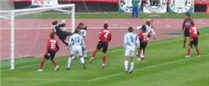# Mutually Exclusive EventsMutually Exclusive: can't happen at the same time.

Examples:

• Turning left and turning right are Mutually Exclusive (you can't do both at the same time)
• Tossing a coin: Heads and Tails are Mutually Exclusive
• Cards: Kings and Aces are Mutually Exclusive

What is not Mutually Exclusive:

• Turning left and scratching your head can happen at the same time
• Kings and Hearts, because we can have a King of Hearts!

Like here:Aces and Kings are Mutually Exclusive (can't be both) Hearts and Kings are not Mutually Exclusive (can be both)

## Probability

Let's look at the probabilities of Mutually Exclusive events. But first, a definition:

Probability of an event happening = Number of ways it can happen Total number of outcomes

### Example: there are 4 Kings in a deck of 52 cards. What is the probability of picking a King?

Number of ways it can happen: 4 (there are 4 Kings)

Total number of outcomes: 52 (there are 52 cards in total)

So the probability = 4 52 = 1 13

## Mutually Exclusive

When two events (call them "A" and "B") are Mutually Exclusive it is impossible for them to happen together:

P(A and B) = 0

"The probability of A and B together equals 0 (impossible)"

### Example: King AND Queen

A card cannot be a King AND a Queen at the same time!

• The probability of a King and a Queen is 0 (Impossible)

But, for Mutually Exclusive events, the probability of A or B is the sum of the individual probabilities:

P(A or B) = P(A) + P(B)

"The probability of A or B equals the probability of A plus the probability of B"

### Example: King OR Queen

In a Deck of 52 Cards:

• the probability of a King is 1/13, so P(King)=1/13
• the probability of a Queen is also 1/13, so P(Queen)=1/13

When we combine those two Events:

• The probability of a King or a Queen is (1/13) + (1/13) = 2/13

Which is written like this:

P(King or Queen) = (1/13) + (1/13) = 2/13

So, we have:

• P(King and Queen) = 0
• P(King or Queen) = (1/13) + (1/13) = 2/13

## Special Notation

Instead of "and" you will often see the symbol (which is the "Intersection" symbol used in Venn Diagrams)

Instead of "or" you will often see the symbol (the "Union" symbol)

So we can also write:

• P(King Queen) = 0
• P(King Queen) = (1/13) + (1/13) = 2/13### Example: Scoring Goals

If the probability of:

• scoring no goals (Event "A") is 20%
• scoring exactly 1 goal (Event "B") is 15%

Then:

• The probability of scoring no goals and 1 goal is 0 (Impossible)
• The probability of scoring no goals or 1 goal is 20% + 15% = 35%

Which is written:

P(A B) = 0

P(A B) = 20% + 15% = 35%

## Remembering"Or has more ... than And"

Also is like a cup which holds more than

## Not Mutually Exclusive

Now let's see what happens when events are not Mutually Exclusive.

### Example: Hearts and KingsHearts and Kings together is only the King of Hearts:But Hearts or Kings is:

• all the Hearts (13 of them)
• all the Kings (4 of them)

But that counts the King of Hearts twice!

So we correct our answer, by subtracting the extra "and" part:16 Cards = 13 Hearts + 4 Kings − the 1 extra King of Hearts

Count them to make sure this works!

As a formula this is:

P(A or B) = P(A) + P(B) − P(A and B)

"The probability of A or B equals the probability of A plus the probability of B
minus the probability of A and B"

Here is the same formula, but using and :

P(A ∪ B) = P(A) + P(B) − P(A ∩ B)

## A Final Example

16 people study French, 21 study Spanish and there are 30 altogether. Work out the probabilities!

This is definitely a case of not Mutually Exclusive (you can study French AND Spanish).

Let's say b is how many study both languages:

• people studying French Only must be 16-b
• people studying Spanish Only must be 21-b

And we get:And we know there are 30 people, so:

(16−b) + b + (21−b) = 30
37 − b = 30
b = 7

And we can put in the correct numbers:So we know all this now:

• P(French) = 16/30
• P(Spanish) = 21/30
• P(French Only) = 9/30
• P(Spanish Only) = 14/30
• P(French or Spanish) = 30/30 = 1
• P(French and Spanish) = 7/30

Lastly, let's check with our formula:

P(A or B) = P(A) + P(B) − P(A and B)

Put the values in:

30/30 = 16/30 + 21/30 − 7/30

Yes, it works!

## Summary:

### Mutually Exclusive

• A and B together is impossible: P(A and B) = 0
• A or B is the sum of A and B: P(A or B) = P(A) + P(B)

### Not Mutually Exclusive

• A or B is the sum of A and B minus A and B: P(A or B) = P(A) + P(B) − P(A and B)

### Symbols

• And is (the "Intersection" symbol)
• Or is (the "Union" symbol)